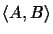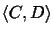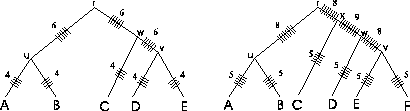Next: Traveling Salesman Up: Methods Previous: Methods

## Sum of Pairs versus Circular Sum measure

The sum of pairs (SP) measure is a well known scoring function for MSAs [3,18,19,25]. We introduce the SP measure to show our motivation to find a better scoring function for MSAs. The connection to evolutionary trees will be clear after the following paragraph. To calculate the score of an MSA with the SP measure , allscores of the pairwise alignments are added up. SP methods are obviously deficient from an evolutionary perspective. Consider a tree (Figure 1) constructed for a family containing five proteins. The score of a pairwise alignmentevaluates the probability of evolutionary events on edges (u, A) and (u, B) of the tree; that is, the edges that represent the evolutionary distance between sequence A and sequence B. Likewise, the score of a pairwise alignmentevaluates the probability of evolutionary events on edges (C, w), (w, v) and (v, D) of the tree. The edge lengths correspond to the PAM distances.By adding ticks'' to the evolutionary tree that are drawn each time an edge is evaluated when calculating the SP score (Figure 1), it is readily seen that with the SP method different edges of the evolutionary tree of the protein family are counted a different numbers of times. In the example tree on the left side, edges (r, u), (r, w) and (w, v) are each counted six times by the SP method, while edges (u, A), (u, B), (v, D), (v, E), and (w, C) are each counted four times (numbers on the edges in Figure 1). It gets worse as the tree grows (see tree on the right). Thus, SP methods are intrinsically problematic from an evolutionary perspective for scoring MSAs. This was the motivation to developed a scoring method that evaluates each edge equally. In addition, we wanted a scoring function for MSAs that does not depend on the actual tree structure. How this can be achieved is explained in the next section.Next: Traveling Salesman Up: Methods Previous: Methods
Chantal Korostensky
1999-07-14